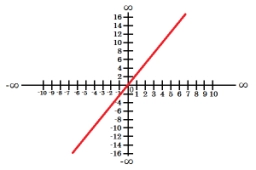# Temperature - linear function

In the morning, at 08:00 hours, the temperature was -8°C, and in the afternoon, at 16:00 hours, the temperature was 24°C. Assuming that the temperature changes steadily, what was the temperature at 1300 hours, and what was the time when the temperature was 8°C?

t3 =  12 °C
T4 =  12 h

### Step-by-step explanation:Did you find an error or inaccuracy? Feel free to write us. Thank you!

Tips for related online calculators
The line slope calculator is helpful for basic calculations in analytic geometry. The coordinates of two points in the plane calculate slope, normal and parametric line equation(s), slope, directional angle, direction vector, the length of the segment, intersections of the coordinate axes, etc.
Do you have a linear equation or system of equations and looking for its solution? Or do you have a quadratic equation?
Do you want to convert time units like minutes to seconds?